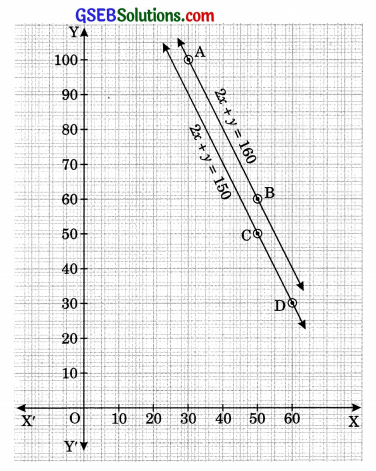# GSEB Solutions Class 10 Maths Chapter 3 Pair of Linear Equations in Two Variables Ex 3.1

Gujarat Board GSEB Solutions Class 10 Maths Chapter 3 Pair of Linear Equations in Two Variables Ex 3.1 Textbook Questions and Answers.

## Gujarat Board Textbook Solutions Class 10 Maths Chapter 3 Pair of Linear Equations in Two Variables Ex 3.1

Question 1.
Aftab tells his daughter, “Seven years ago, I ‘ was seven times as old as you were then. Also, three years from now, I shall be three times as old as you will be.” (Isn’t this interesting?) Represent this situation algebraically and graphically.
Solution:
Let the present age of Aftab be x years and the present age of his daughter be y years. Algebraic representation of the given situation is
x – 7 = 7(y – 7)
and x + 3 = 3(y + 3)
⇒ x – 7y + 42 = 0    …(1)
x – 3y – 6 = 0 …(2)
Graphical representation, we find two solutions for each equation.
For equation (1)
x – 7y + 42 = 0
x = 7y – 42Table 1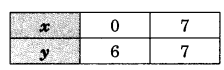For equation (2)
x – 3y – 6 = 0
x = 3y + 6
Table 2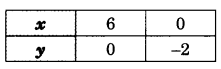We plot the points A(0, 6) and B(7, 7) corresponding to the solutions in table 1 on a graph paper to get the line AB representing the equation (1) and the points C(6, 0) and D(0, -2) on the same graph paper to get the line CD representing the equation (2).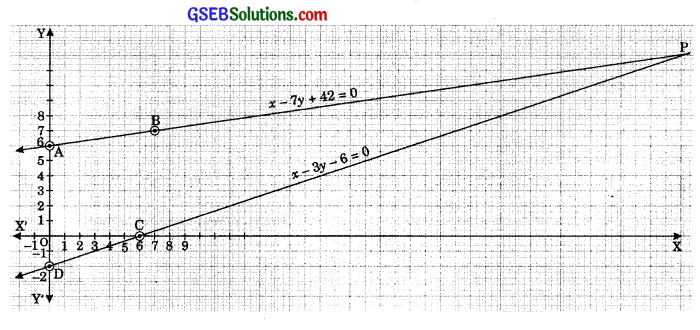Question 2.
The coach of a cricket team buys 3 bats and 6 balls for ₹ 3900. Later, she buys another bat and 3 more balls of the same kind for ₹ 1300. Represent this situation algebraically and geometrically.
Solution:
Let the cost of one bat be ₹ x
and the cost of one ball be ₹ y.
Then, the algebraic representation of the given situation is
3x + 6y = 3900
and x + 3y = 1300
⇒ x + 2y = 1300 …(1)
x + 3y = 1300 …(2)For graphical representation, we find two solutions for each equation.
For equation (1)
x + 2y = 1300
x = 1300 – 2y
Table 1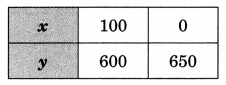For equation (2)
x + 3y = 1300
x = 1300 – 3y
Table 2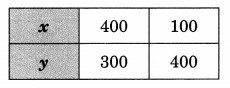We plot the points A(100, 600) and B(0, 650) corresponding to the solutions in table 1 on graph paper to get the line AB representing the equation (1) and the points C(400, 300) and D(100, 400) corresponding to the solutions in table 2 on the same graph paper to get the line CD representing the equation (2).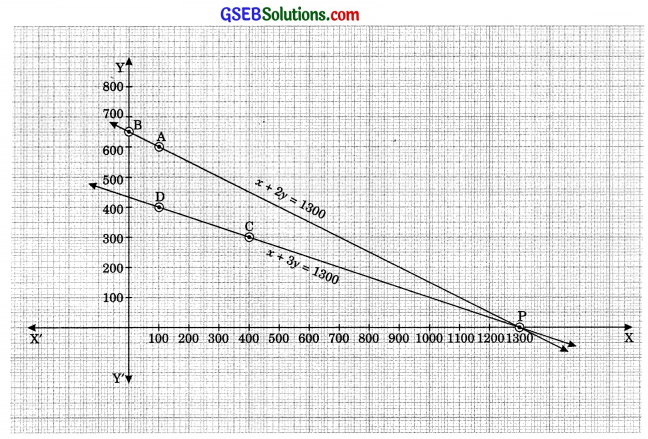Question 3.
The cost of 2 kg of apples and 1 kg of grapes on a day was found to be ₹ 160. After a month, the cost of 4 kg of apples and 2 kg of grapes is ₹ 300. Represent the situation algebraically and geometrically.
Solution:
Let the cost of 1 kg of apples be ₹ x
and the cost of 1 kg of grapes be ₹ y.
Algebraic representation of the given situation is
2x + y = 160 …(1)
4x + 2y = 300
⇒ 2x + y = 150 …(2)
For graphical representation we find two solutions for each equation.
For equation (1)
2x + y = 160
y = 160 – 2xTable 1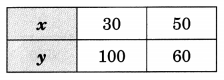For equation (2)
2x + y = 150
y = 150 – 2x
Table 2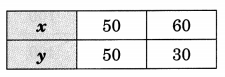We plot the points A(30, 100) and B(50, 60) corresponding to the solutions in table 1 on a graph paper to get the line AB representing the equation (1) and the points C(50, 50) and D(60, 30) corresponding to the solutions in table 2 on the same graph paper to get the line CD representing the equation (2).